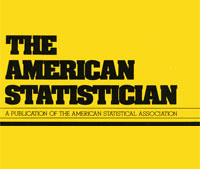## more concentration, everywhere

Posted in R, Statistics with tags , , , , , , , , , , on January 25, 2019 by xi'anAlthough it may sound like an excessive notion of optimality, one can hope at obtaining an estimator δ of a unidimensional parameter θ that is always closer to θ that any other parameter. In distribution if not almost surely, meaning the cdf of (δ-θ) is steeper than for other estimators enjoying the same cdf at zero (for instance ½ to make them all median-unbiased). When I saw this question on X validated, I thought of the Cauchy location example, where there is no uniformly optimal estimator, albeit a large collection of unbiased ones. But a simulation experiment shows that the MLE does better than the competition. At least than three (above) four of them (since I tried the Pitman estimator via Christian Henning’s smoothmest R package). The differences to the MLE empirical cd make it clearer below (with tomato for a score correction, gold for the Pitman estimator, sienna for the 38% trimmed mean, and blue for the median):I wonder at a general theory along these lines. There is a vague similarity with Pitman nearness or closeness but without the paradoxes induced by this criterion. More in the spirit of stochastic dominance, which may be achievable for location invariant and mean unbiased estimators…

## an improvable Rao–Blackwell improvement, inefficient maximum likelihood estimator, and unbiased generalized Bayes estimator

Posted in Books, Statistics, University life with tags , , , , , , , , on February 2, 2018 by xi'anIn my quest (!) for examples of location problems with no UMVU estimator, I came across a neat paper by Tal Galili [of R Bloggers fame!] and Isaac Meilijson presenting somewhat paradoxical properties of classical estimators in the case of a Uniform U((1-k)θ,(1+k)θ) distribution when 0<k<1 is known. For this model, the minimal sufficient statistic is the pair made of the smallest and of the largest observations, L and U. Since this pair is not complete, the Rao-Blackwell theorem does not produce a single and hence optimal estimator. The best linear unbiased combination [in terms of its variance] of L and U is derived in this paper, although this does not produce the uniformly minimum variance unbiased estimator, which does not exist in this case. (And I do not understand the remark that

“Any unbiased estimator that is a function of the minimal sufficient statistic is its own Rao–Blackwell improvement.”

as this hints at an infinite sequence of improvement.) While the MLE is inefficient in this setting, the Pitman [best equivariant] estimator is both Bayes [against the scale Haar measure] and unbiased. While experimentally dominating the above linear combination. The authors also argue that, since “generalized Bayes rules need not be admissible”, there is no guarantee that the Pitman estimator is admissible (under squared error loss). But given that this is a uni-dimensional scale estimation problem I doubt very much there is a Stein effect occurring in this case.

## best unbiased estimators

Posted in Books, Kids, pictures, Statistics, University life with tags , , , , , , , , , , , , on January 18, 2018 by xi'anA question that came out on X validated today kept me busy for most of the day! It relates to an earlier question on the best unbiased nature of a maximum likelihood estimator, to which I pointed out the simple case of the Normal variance when the estimate is not unbiased (but improves the mean square error). Here, the question is whether or not the maximum likelihood estimator of a location parameter, when corrected from its bias, is the best unbiased estimator (in the sense of the minimal variance). The question is quite interesting in that it links to the mathematical statistics of the 1950’s, of Charles Stein, Erich Lehmann, Henry Scheffé, and Debabrata Basu. For instance, if there exists a complete sufficient statistic for the problem, then there exists a best unbiased estimator of the location parameter, by virtue of the Lehmann-Scheffé theorem (it is also a consequence of Basu’s theorem). And the existence is pretty limited in that outside the two exponential families with location parameter, there is no other distribution meeting this condition, I believe. However, even if there is no complete sufficient statistic, there may still exist best unbiased estimators, as shown by Bondesson. But Lehmann and Scheffé in their magisterial 1950 Sankhya paper exhibit a counter-example, namely the U(θ-1,θ-1) distribution:since no non-constant function of θ allows for a best unbiased estimator.

Looking in particular at the location parameter of a Cauchy distribution, I realised that the Pitman best equivariant estimator is unbiased as well [for all location problems] and hence dominates the (equivariant) maximum likelihood estimator which is unbiased in this symmetric case. However, as detailed in a nice paper of Gabriela Freue on this problem, I further discovered that there is no uniformly minimal variance estimator and no uniformly minimal variance unbiased estimator! (And that the Pitman estimator enjoys a closed form expression, as opposed to the maximum likelihood estimator.) This sounds a bit paradoxical but simply means that there exists different unbiased estimators which variance functions are not ordered and hence not comparable. Between them and with the variance of the Pitman estimator.